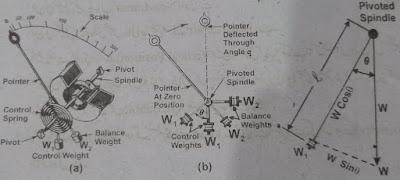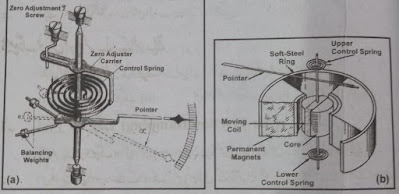# Deflecting Force-Controlling Force-Damping Force-Instrument Forces-Eddy Current

This article describes the three forces (Deflecting Force, Damping Force, and Controlling Force) that are used on instruments. Also, detailed information about Gravity Control Method and Spring Control Method is given. Here are the advantages and disadvantages of all forces.

# Deflecting Force

Deflecting force is a force used to move or move an instrument's moving system from its stationary (zero) position. The amount of force can vary in different instruments. It depends on the design type of the drinking device and the amount of friction that occurs on the moving system and the required sensitivity and accuracy.

The deflecting force is generated by one of the following effects of the current.

• Magnetic effect
• Heating Effect
• Electrostatic effect
• Electromagnetic effect

## Controlling Force

A controlling force is a force that is used to control the moving stem of an instrument after moving it to a certain position. This force acts in the opposite direction to the deflecting force. As the deflecting force increases, so does the controlling force. The pointer stops where the two forces are equal.

Deflecting Torque (Td) = Controlling Torque (Tc)

The controlling force is also necessary to bring the pointer back to zero position. When the supply of the instrument is cut off and the deflecting force is exhausted. That's it. Their explanation is coming up.

• Gravity Control Method (GCM)
• Spring Control Method (SCM)

## Ways to get the Controlling Force

### 1. Gravity Control Method (GCM)

When weight is lifted above the ground, the gravity of the earth pulls it back. This principle is used in Indicating Instruments to provide control torque. That is why this method is called Gravity Control Method.

This method uses a small control weight to gain controlling force. For this, two wide arms are made on the back of the pointer. One nut is placed on each arm as a weight (Sometimes a second nut is used to make the lock nut). One of these weights, W1 acts as a controlling weight while the other weight is used to balance the W2 moving system. The control torque value can be changed by moving the control weight, W1 back and forth on the wide arm.

When the pointer is at zero position, the control weight is in the vertical position. As the instrument's moving system moves with the pointer, the weight, “W1” also begins to rise at an angle" 0 "in the clockwise direction, as shown by the figure. At this point, the gravity of the earth pulls this weight towards itself and provides controlled torque.Gravity Method

Vector W splits into two components. One is horizontal, WCosѲ and the other is vertical, WsinѲ. The control force is proportional to the sine of the deflection angle. If the arm length is "l" then the control torque is "Tc".

Tc = WlsineѲ     or   Tc α Sin Ѳ…. (1)

If the deflecting torque is directly proportional to the current. The pointer reading is static when the two torques are equal. The current is directly proportional to the sine of the deflection angle. Therefore the scales of these instruments are also uneven.

### Advantages of Gravity Control System (GCS)

Gravity control instruments have the following advantages over spring control instruments.

• It is very simple and cheap.
• Not affected by temperature changes.
• Their performance is not affected by the passage of time.
• It can be adjusted very easily.
• Adjusting the control torque does not require much skill.

### Disadvantages of Gravity Control System (GCS)

• Their scale is non-linear.
• Their scale cannot be made too long, it is limited to 80° only.
• They are used only in the Vertical Position so that the controls can carry the weight.
• Always pay special attention to the level during use so that the zero position is not affected. (Bubble level is used in such instruments for this purpose.)
• Controlling weight increases the weight of the moving system, which gives the impression of sensitivity of the instrument.

### Spring Control Method (SCM)

In this method, a spring is used to gain controlling force. Moving iron instruments use a strip spiral spring on the upper side of the spindle while moving coil instruments use two ball contact springs (one on the upper side of the spindle and one on the lower side).

Hair springs are usually made of phosphor bronze material (also silicon bronze, platinum silver, platinum radium, German silver, etc.). One end of the spring is soldered to the spindle of the instrument while the other end is soldered to a stationary part of the instrument. As the instrument's moving system begins to move, the spring begins to twist or compress around the spindle in the opposite direction. This produces anti-controlling or restoring torque inside the spring which is directly proportional to the deflecting angle "0" of the moving system. Therefore, the scale of spring-controlled instruments is the same.

Where the amounts of deflecting torque "Td" and controlling torque "Tc" are equal. This is where the pointer stops. When the deflecting force is exhausted (or reduced to zero), the spring returns to its original position. This is how the pointer returns to its zero position.Instruments Forces

The springs have been shown solely to give a sense of proportion. The spring should not be pressurized so much that the shape of the spring changes permanently. The change in temperature affects the length of the spring which affects the deflection. Therefore if the spindle prawns were wrapped in two identical springs in opposite directions then the change in their lengths due to temperature would be uniform. Is. This way there is no effect on the deflection.

### Advantages of Spring Control Method (SCM)

• They are very lightweight and small in size.
• Levels do not have to be taken care of while using.
• Their scale is the same.
• Spring loses its weight. Therefore the weight of the moving system does not increase.

## Damping Force

In electronic measuring instruments, the pointer does not immediately stop at the final position but keeps swinging there due to stagnation. Which wastes time in taking readings. And the readings are not accurate either. Therefore, the force used to stop the pointer at the final position without swinging or dead beat is called damping force. This force acts when the moving system is moving. It is always in the opposite direction of movement of the moving system.

The following three methods are used to provide damping force in indicating instruments.

• Air friction
• Fluid Fraction Damping
• Eddy Current Damping

Eddy Current